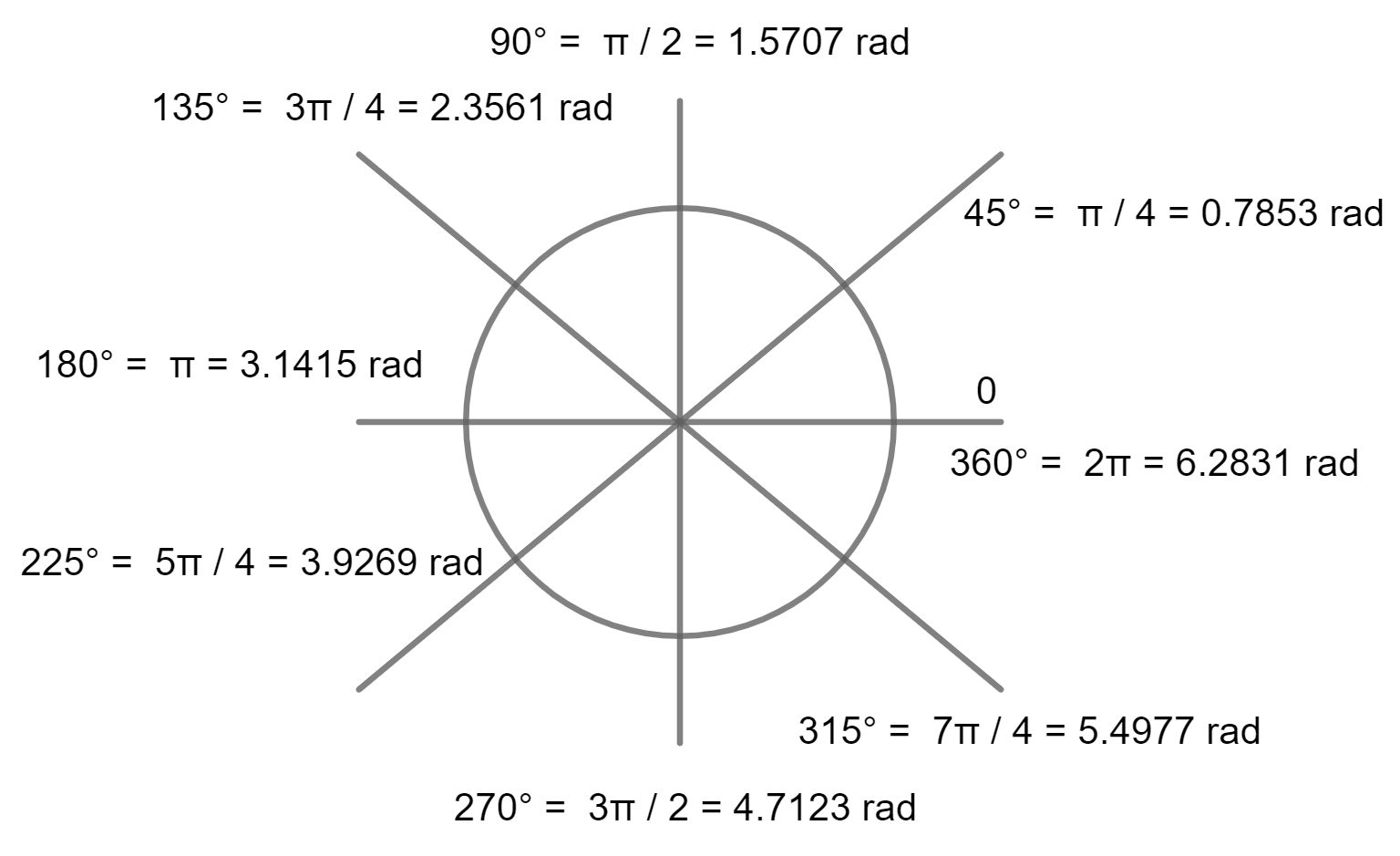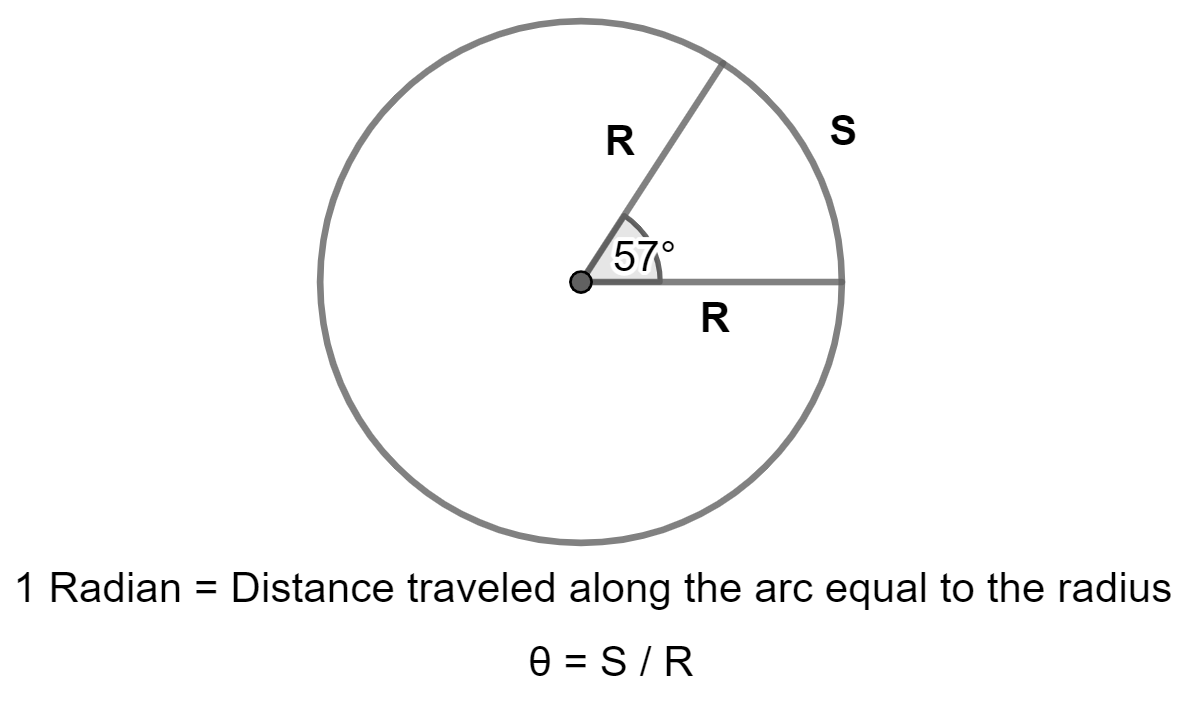Home

Converter

=

Enter either radian or degree and calculate the equivalent

## How to convert Degree to Radians. How Many Degrees In Radians?• ### 1 ° = 0.01745329252 rad

• For example, to convert 90 degrees to radians, you would use the following formula:
• radians = 90° * π / 180°

Therefore,

### 90 degrees is equal to π / 2 radians.

• There are 6.28 (2 * 3.14) radians in a circle (radian unit circle).
• There are 3.14 radians in half circle.

• ### 1 rad = 57.295779513 ° (1 radian is 57.29 degrees)

• Radians × 180 / π
• For example, to convert 0.5 radians to degrees, you would use the following formula:
• degrees = 0.5 * 180 / π
• This would give you a result of 0.5 radians = 28.65 degrees.

## Introduction to Radians and Degrees• ### The central angle of 1 radian subtends an arc length that is equal to the radius of the circle.

This means that 1 radian is equal to 57.2958 degrees.

In other words, a radian angle measure is 57 degrees
• Angles are measured in degrees. One revolution is divided into 360 equal parts and each part is known as a degree.

• ### The unit circle is a circle with a radius of 1 unit

. An angle of 1 radian subtends an arc length of 1 unit on the unit circle. This makes the unit circle a convenient tool for visualizing angles in radians.• ### The radian is the natural unit of angle measurement

. This is because it is based on the circumference of a circle, which is a fundamental geometric concept. Degrees, on the other hand, are an arbitrary unit of angle measurement that is based on the number of days in a year.
• ### Degrees are the more common unit of measurement,

and are used in everyday life and in many industries, such as construction and engineering.
• Degrees are often used in measuring angles on a compass or when setting the time on a clock.
• Degrees are not as precise as radians and can lead to errors when performing calculations but they are easier to visualize, more common and intuitive.
• Radians are difficult to visualize but more precise and therefore more convenient to use in mathematics and physics and in computer graphic applications. Many trigonometric functions, such as sine and cosine, are defined in terms of radians.
• The radian is named after the French mathematician François Viète. Viète was a pioneer in the use of trigonometric functions in mathematics.
• The degree is named after the Greek astronomer Hipparchus. Hipparchus was the first person to divide the circle into 360 degrees.
• The radian is the preferred unit of angle measurement in the International System of Units (SI).
• The degree is still widely used in many fields, such as engineering, surveying, and navigation.
• Which unit of measurement is better depends on the specific application. For everyday use, degrees are generally the better choice. For mathematical and physical calculations, radians are generally the better choice.
Here are some examples of when to use degrees and radians:
• Degrees:
• Measuring the angle of a turn on a steering wheel.
• Measuring the angle of a roof pitch.
• Measuring the angle of a mountain slope.
• Calculating the circumference of a circle.
• Calculating the area of a sector.
• Calculating the velocity of an object moving in a circular path.

Note: This content has inputs from both the AI language model developed by OpenAI and by Bard, the Google AI model.### Angle in Degree (deg)### In Degrees (deg)

#### 1800 degrees (Five Circle)